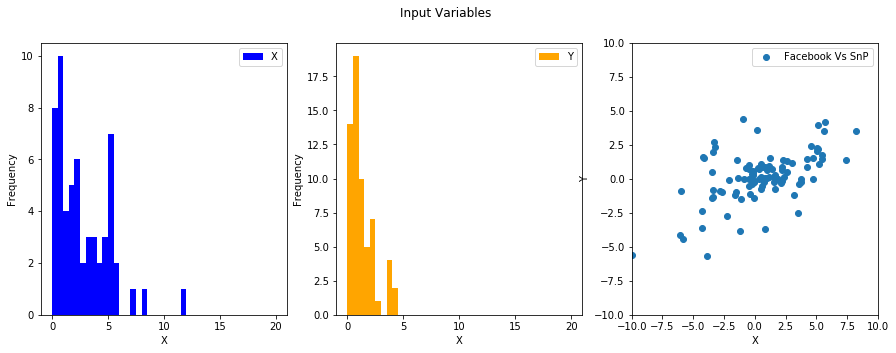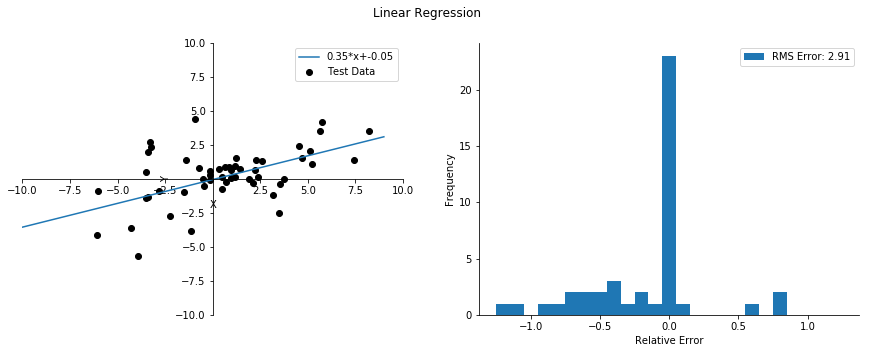Back To Index

04_LinearRegression_Scikit

In this exercise, we will build upon what we learned in previous sections to build a regression model using a realistic dataset. We will read the data from a CSV file into two parallel lists X and Y. Thereafter we setup a linear regression using X as independent variable and Y as dependent.

### Dataset¶

The CSV file contains three columns namely ‘DDate’, ‘FB’ and ‘SNP’. The ‘DDate’ is the first day of week, ‘FB’ and ‘SNP’ are the weekly returns for Facebook stock and S&P-500 index respectively.  DDate,FB,SNP 2017-06-12,1.667,0.2997 2017-06-19,2.2148,-0.174 2017-06-26,-3.3728,-0.8149 2017-07-03,-0.1846,-0.2554 2017-07-10,5.4585,1.4337 2017-07-17,2.6084,0.5302 

### Analysis Outlook¶

The S&P 500 Index is a combined metric of stock market’s performance constructed by averaging 500 largest U.S. publicly traded companies weighted by market-capitalization. The index is widely regarded as the best gauge of large-cap U.S. equities. We will test this persumption by comparing the weekly returns on S&P 500 to those on Facebook’s stock.

In :
#############################################
# Filename: linear_regression_csvfile       #
# Purpose: A simple demonstration of        #
# python program structure.                 #
# Author : Niel S.                          #
#  The English Tea Company LLC.             #
#############################################

from matplotlib import pyplot as plt
from sklearn import linear_model, metrics

#The Desktop
desktop = 'C:\\Users\\singa72\\Desktop\\'
data   = desktop+'returns_fb_snp.csv'

def main():
#Visualizing the data.
visualize_input_data(X,Y)
#Obtain the coefficients of regression
X_t,Y_t,Y_mdl,A, B = linear_regression(X,Y)
#Let us visualize the model's performance
visualize_trained_model(X_t,Y_t,Y_mdl,A,B)

plt.show()

'''
Input Parameters:
data_file : Fully qualified name for data file.
Returns:
Parallel lists
X : Weekly return on Facebook stock
Y : Weekly return on S&P500 index
'''
file = open(data_file)
X,Y = [],[]
for line in file:
line = line.replace('\n','')
#print ('Line Before Splitting: ',line)
line = line.split(',')
#print ('Line After Splitting: ',line)

if len(line) < 3:
continue

else:
X.append(float(line))
Y.append(float(line))
file.close()
return X,Y

def linear_regression(X,Y):
'''
Input Parameters:
X : 1D list of independent values.
Y : 1D list of dependent values.
Returns:
Parallel Lists
X_test : Facebook Returns test set
Y_test : S&P 500 Returns test set
Y_mdl  : S&P 500 Regression value.
Numeric values:
a : Parameter a of regression line ax+b
b : Parameter b of regression line ax+b
'''
#Change the row format [a,b,c] to column format [[a],[b],[c]]
X_train =[[x] for x in X[0:50] ]
X_test  =[[x] for x in X[50:]  ]
Y_test  =['Exercise: Add Code Here' ]

#Creating an instance of LinearRegression
reg = linear_model.LinearRegression()

#Train the model using training sets.

#Use test set to make predictions.
Y_mdl = reg.predict(X_test).tolist()

#The slope and The Intercept
a = reg.coef_
b = reg.intercept_

#Restore back from column format to row format.
X_test = [x for x in X_test]
Y_test = [y for y in Y_test]
Y_mdl  = [y for y in Y_mdl]

return X_test,Y_test,Y_mdl,a,b

def visualize_trained_model(X_test,Y_test,Y_mdl,A,B):
'''
Publish a 2-D scatterplot between
X and Y overlaid with the straight
line : A*x+B = Y
'''
#Create a figure object
fig0,(ax0,ax1) = plt.subplots(1,2,figsize=(15,5))
##Do a scatter plot for the variables.
ax0.scatter (X_test,Y_test,color='black',label='Test Data')

#Set axes labels
ax0.set_xlabel('X')
ax0.set_ylabel('Y')

#Set axes ranges
ax0.set_xlim([-10,10])
ax0.set_ylim([-10,10])

##Now is the time to generate the regression line.
A = round(A,2)
B = round(B,2)
X_line = [i for i in range(-10,10)]
Y_line = [A*x+B for x in X_line]
ax0.plot(X_line,Y_line,label = str(A)+'*x'+'+'+str(B))
#Erase the verticle frame spines
ax0.spines['right'].set_color('none')
ax0.spines['top'].set_color('none')
#Move the remaining spines to center.
ax0.spines['left'].set_position('center')
ax0.spines['bottom'].set_position('center')
ax0.set_xticks([-10,-7.5,-5,-2.5,2.5,5,7.5,10])
ax0.set_yticks([-10,-7.5,-5,-2.5,2.5,5,7.5,10])

#Draw Legend
ax0.legend()

##Let us look at the modeling errors: residuals.
Y_errors = [(y_mdl-y_test)/y_test  if y_test > 0 else 0 for y_test,y_mdl in zip(Y_test, Y_mdl)]
Y_errors_rms = round ( sum([e*e for e in Y_errors]) / len(Y_errors),2)

##A histogram of the residuals.
err_min,err_max,binw = -1.25,1.25,0.1
nbins = int ((err_max-err_min)/binw)+1
bins  = [err_min+i*binw for i in range(0,nbins)]
ax1.hist(Y_errors,bins=bins,label='RMS Error: '+str(Y_errors_rms))

#Set axes labels
ax1.set_xlabel('Relative Error')
ax1.set_ylabel('Frequency')
#Erase the spines
ax1.spines['right'].set_color('none')
ax1.spines['top'].set_color('none')
#Draw the legend
ax1.legend()

# Explained variance score: 1 is perfect prediction
print('Variance score: %.2f'% metrics.r2_score(Y_test, Y_mdl))

fig0.suptitle('Linear Regression')

def visualize_input_data(X,Y):
'''
Input:
X : a column of x values.
Y : a column of y values.
'''

#Create a figure object with 3 axes.
fig0,(ax0,ax1,ax2) = plt.subplots(1,3,figsize=(15,5))

#How many bins we need for histograming?
max_range = 10
min_range = -10
bin_width = 0.5
####Number of bins
nbins =int((max_range-min_range)/bin_width)
####Bins
bins = [0+i*bin_width for i in range(0,nbins+1)]

#Create the histogram a.k.a frequency chart of X
n0,b0,p0 = ax0.hist(X,bins=bins,histtype='stepfilled',label='X',color='blue')

#Create a histogram of Y (same binning as in X).
n1,b1,p1 = ax1.hist(Y,bins=bins,histtype='stepfilled',label='Y',color='orange')

#Some Cosmetic settings for ax0
ax0.set_xlabel('X')
ax0.set_ylabel('Frequency')
ax0.legend()

#Some Cosmentics settings for ax1
ax1.set_xlabel('X')
ax1.set_ylabel('Frequency')
ax1.legend()

##Do a scatter plot for the variables.

#Set axes labels
ax2.set_xlabel('X')
ax2.set_ylabel('Y')

#Set axes ranges
ax2.set_xlim([-10,10])
ax2.set_ylim([-10,10])

#Display the legend
ax2.legend()

#Set a figure title

fig0.suptitle('Input Variables')

if __name__ == '__main__':
main()

DDate,FB,SNP

Variance score: 0.20Back To Index